## How to Convert Square Miles to Acres | Area Units

The image below represents square miles to acres.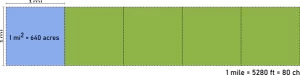To convert square miles to acres, one essential parameter is needed and this parameter is Unit Value.

The formula for converting square miles to acres:

1 Square Mile = 640 Acres

Let’s solve an example;
Find the conversion of square miles to acres when the unit value is 4.

1 Square Mile = 640 Acres

Then,

4 Square Miles = (4 x 640) Acres
4 Square Miles = 2560 Acres

Therefore, the acre(s) is 2560.

## How to Convert Acres to Square Miles | Area Units

The image below represents acres to square miles.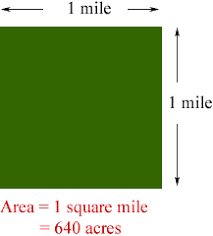To convert acres to square miles, one essential parameter is needed and this parameter is Unit Value.

The formula for converting acres to square miles:

1 Acre = 0.001563 Square Miles

Let’s solve an example;
Find the conversion of acres to square miles when the unit value is 12.

1 Acre = 0.001563 Square Miles

Then,

12 Acres = (12 x 0.001563) Square Miles
12 Acres = 0.018756 Square Miles

Therefore, the square mile(s) is 0.018756.

## How to Convert Acres to Square Yards | Area Units

The image below represents acres to square yards.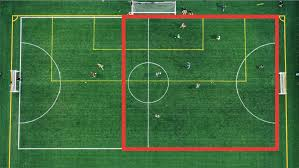To convert acres to square yards, one essential parameter is needed and this parameter is Unit Value.

The formula for converting acres to square yards:

1 Acre = 4840 Square Yards

Let’s solve an example;
Find the conversion of acres to square yards when the unit value is 10.

1 Acre = 4840 Square Yards

Then,

10 Acres = (10 x 4840) Square Yards
10 Acres = 48400 Square Yards

Therefore, the square yard(s) is 48400.

## How to Convert Acres to Square Feet | Area Units

The image below represents acres to square feet.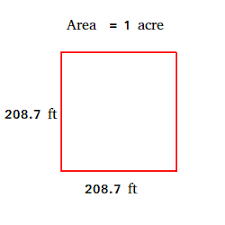To convert acres to square feet, one essential parameter is needed and this parameter is Unit Value.

The formula for converting acres to square feet:

1 Acre = 43560 Square Feet

Let’s solve an example;
Find the conversion of acres to square feet when the unit value is 9.

1 Acre = 43560 Square Feet

Then,

9 Acres = (9 x 43560) Square Feet
9 Acres = 392040 Square Feet

Therefore, the square feet is 392040.

## How to Convert Acres to Square Inches | Area Units

The image below represents acres to square inches.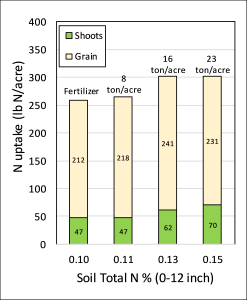To convert acres to square inches, one essential parameter is needed and this parameter is Unit Value.

The formula for converting acres to square inches:

1 Acre = 627640 Square Inches

Let’s solve an example;
Find the conversion of acres to square inches when the unit value is 8.

1 Acre = 627640 Square Inches

Then,

8 Acres = (8 x 6276640) Square Inches
8 Acres = 50213120 Square Inches

Therefore, the square inch(es) is 50213120.

## How to Convert Acres to Kilometers | Area Units

The image below represents acres to square kilometers.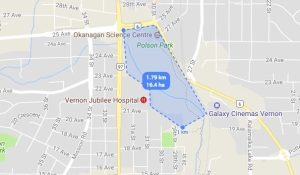To convert acres to square kilometers, one essential parameter is needed and this parameter is Unit Value.

The formula for converting acres to square kilometers:

1 Acre = 0.004047 Square Kilometers

Let’s solve an example;
Find the conversion of acres to square kilometers when the unit value is 7.

1 Acre = 0.004047 Square Kilometers

Then,

7 Acres = (7 x 0.004047) Square Kilometers
7 Acres = 0.028329 Square Kilometers

Therefore, the square kilometer(s) is 0.028329.

## How to Convert Acres to Hectares | Area Units

The image below represents acres to hectares.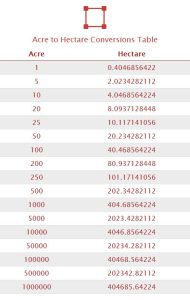To convert acres to hectares, one essential parameter is needed and this parameter is Unit Value.

The formula for converting acres to hectares:

1 Acre = 0.404686 Hectares

Let’s solve an example;
Find the conversion of acres to hectares when the unit value is 6.

1 Acre = 0.404686 Hectares

Then,

6 Acres = (6 x 0.404686) Hectares
6 Acres = 2.428116 Hectares

Therefore, the hectare(s) is 2.428116.

## How to Convert Acres to Square Meters | Area Units

The image below represents acres to square meters.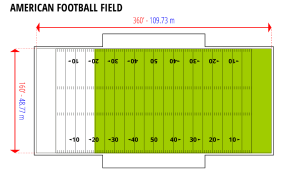To convert acres to square meters, one essential parameter is needed and this parameter is Unit Value.

The formula for converting acres to square meters:

1 Acre = 4046.856 Square Meters

Let’s solve an example;
Find the conversion of acres to square meters when the unit value is 5.

1 Acre = 4046.856 Square Meters

Then,

5 Acres = (5 x 4046.856) Square Meters
5 Acres = 20234.280000000002 Square Meters

Therefore, the square meter(s) is 20234.2

## How to Convert Acres to Square Centimeters | Area Units

The image below represents acres to square centimeters.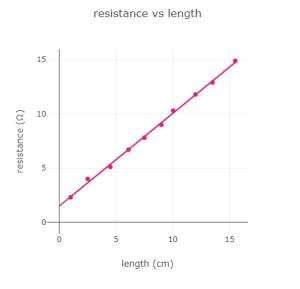To convert acres to square centimeters, one essential parameter is needed and this parameter is Unit Value.

The formula for converting acres to square centimeters:

1 Acre = 40468564 Square Centimeters

Let’s solve an example;
Find the conversion of acres to square centimeters when the unit value is 4.

1 Acre = 40468564 Square Centimeters

Then,

4 Acres = (4 x 40468564) Square Centimeters
4 Acres = 161874256 Square Centimeters

Therefore, the square centimeter(s) is 161874256.

## How to Convert Acres to Square Millimeters | Area Units

The image below represents acres to square millimeters.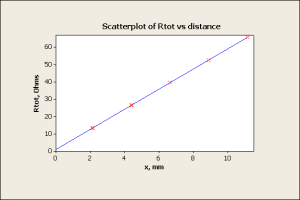To convert acres to square millimeters, one essential parameter is needed and this parameter is Unit Value.

The formula for converting acres to square millimeters:

Let’s solve an example;
Find the conversion of acres to square millimeters when the unit value is 3.

1 Acre = 4046856422 Square Millimeters

Then,

3 Acres = (3 x 4046856422) Square Millimeters
3 Acres = 12140569266 Square Millimeters

Therefore, the square millimeter(s) is 12140569266.